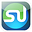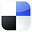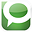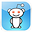# Normal DistributionCreating an InteractiveGraph in Excel

If you are doing statistical analysis of your marketing program, you will eventually want to plot some statistically-distributed data in an Excel graph. The most commonly-occurring statistical distribution in nature and, of course, in business, is the normal distribution.

Click On Image To See Enlarged View

This blog post and video will show you how to create a user-interactive graph of the normal distribution in Excel. The user of your graph will be able to vary the two parameters of the Normal Distribution - the mean and standard deviation - and then watch the changes instantly reflected in the Excel graph. Note the differences between the graph above and the graph below as a result of changes in the 2 user inputs of mean and standard deviation.

Click On Image To See Enlarged View

We’ll even this one step further. Sometimes you need to show a different between regions under the Normal curve. This article and attached video also provide step-by-step instructions on how to graph the Normal Distribution so that the outer 2% tails colored differently so they will stand out from the rest of the graph. After watching the video, you will be able to easily differentiate any region under the Normal curve by making it a different color.

Here is a step-by-step video showing how to create a user-interactive Excel graph of the Normal Distribution:

The concepts covered in this article really need to be observed in the video for full comprehension. Below is an outline of the major steps appearing in the video that are needed to construct a user-interactive graph in Excel of the Normal Distribution with highlighted outer tails.

1) Create the X-Axis

Click On Image To See Enlarged View

You’ll notice on the video that when the user varies the mean or standard deviation inputs, it is the X-Axis that changes, not the graph. The graph will always show 3 standard deviations of normal distribution from the mean in both directions. It is the X-Axis increments that will change as a result of changes made by the user to the mean and standard deviation. The initial left-hand column starts at the top at -30 and finishes at the bottom at +30. This creates the basis of the 3 standard deviations of Normal Distribution that will show at all times on each side of the mean, regardless of the mean and standard deviation that the user has input.

2) Create 2 columns that will calculate the Normal Distribution’s PDF at each point of the X-Axis.

PDF is the Probability Density Function of the Normal Distribution. The data in each of these 2 columns are the Y values of two identical graphs, one sitting on top of the other.

Click On Image To See Enlarged View

3) Zero out the Y-Values on the outer edge(s) of the 2nd column.

Click On Image To See Enlarged View

The 2nd column sits directly on top of the 1st column. If you set any the Y-values for that graph to 0, you allow the identical graph directly underneath to show through. For example, if you zero out the outer 2% of the Y-values on top and bottom of the 2nd column, you are allowing the lower graph to show through for the outer 2% of each tail. You are, in effect, graphing the outer 2% of the Normal Distribution by deleting the outer 2% of the tails in the upper graph, letting the different-colored lower graph to show.

4) Set the color of all elements on the Excel chart as you see best.

Click On Image To See Enlarged View

For example, you might want to change the color of the upper or lower graphs, or the color of the background. You simply left-click on the element that you wish to change the color of and then select the color in the color dialogue box that pops up. The video shows an example of this being done.

That sums up the steps you’ll see in the video.

Here are links to other training videos of how to create interactive graphs in Excel of some of the other major statistical distributions:

How to Graph the Normal Distribution's Probability Density Function in Excel

How To Graph the Normal Distribution's Cumulative Distribution Function in Excel

How To Graph the Students t Distributions' Probability Density Function in Excel

How To Graph the Chi-Square Distribution's Probability Density Function in Excel

How To Graph the Weibull Distribution's PDF and CDF - in Excel

If You Like This, Then Share It...Excel Master Series Blog Directory

Statistical Topics and Articles In Each Topic

• Histograms in Excel
• Bar Chart in Excel
• Combinations & Permutations in Excel
• Normal Distribution in Excel
• t-Distribution in Excel
• Binomial Distribution in Excel
• z-Tests in Excel
• t-Tests in Excel
• Hypothesis Tests of Proportion in Excel
• Chi-Square Independence Tests in Excel
• Chi-Square Goodness-Of-Fit Tests in Excel
• F Tests in Excel
• Correlation in Excel
• Pearson Correlation in Excel
• Spearman Correlation in Excel
• Confidence Intervals in Excel
• Simple Linear Regression in Excel
• Multiple Linear Regression in Excel
• Logistic Regression in Excel
• Single-Factor ANOVA in Excel
• Two-Factor ANOVA With Replication in Excel
• Two-Factor ANOVA Without Replication in Excel
• Creating Interactive Graphs of Statistical Distributions in Excel
• Solving Problems With Other Distributions in Excel
• Optimization With Excel Solver
• Chi-Square Population Variance Test in Excel
• Analyzing Data With Pivot Tables
• SEO Functions in Excel
• Time Series Analysis in Excel